Courses

# RD Sharma Solutions - Ex-16.4, Congruence, Class 7, Math Class 7 Notes | EduRev

## RD Sharma Solutions for Class 7 Mathematics

Created by: Abhishek Kapoor

## Class 7 : RD Sharma Solutions - Ex-16.4, Congruence, Class 7, Math Class 7 Notes | EduRev

The document RD Sharma Solutions - Ex-16.4, Congruence, Class 7, Math Class 7 Notes | EduRev is a part of the Class 7 Course RD Sharma Solutions for Class 7 Mathematics.
All you need of Class 7 at this link: Class 7

#### Question 1:

Which of the following pairs of triangles are congruent by ASA condition?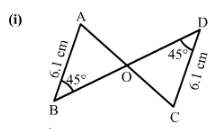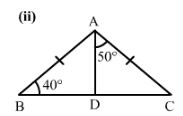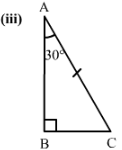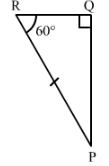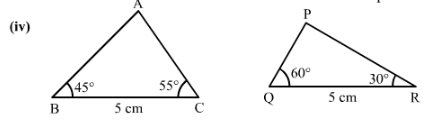1)  We have

Since ∠ABO = ∠CDO = 45° and both are alternate angles,
AB∥DC
∠BAO = ∠DCO  (alternate angle , AB∥CD and AC is a transversal line)
∠ABO = ∠CDO = 45° (given in the figure)
Also, AB = DC    (Given in the figure)
Therefore, by ASA △AOB ≅△DOC

2)
In △ABC ,
Now AB = AC (Given)
∠ABD = ∠ACD = 40° (Angles opposite to equal sides)
∠ABD +∠ACD+∠BAC=180° (Angle sum property)
40°+40°+∠BAC=180°
∠BAC=180°-80°=100°

3)
InΔABC,
∠A+∠B+∠C=180°(Angle sum property)
∠C=180°-∠A-∠B
∠C=180°-30°-90°=60°
InΔPQR,
∠P+∠Q+∠R=180°(Angle sum property)
∠P=180°-∠Q-∠R
∠P=180°-60°-90°=30°
∠BAC = ∠QPR = 30°
∠BCA=∠PRQ = 60°
and AC = PR (Given)
Therefore, by ASA, △ABC ≅△PQR

4)
We have only BC =QR  but none of the angles of △ABC AND △PQR are equal.
Therefore, △ABC≆ △PRQ

#### Question 2:

(ii) State the three pairs of matching parts you have used in (i).
(iii) Is it true to say that BDDC?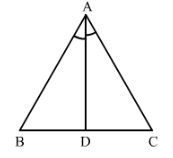#### Question 3:

Draw any triangle ABC. Use ASA condition to construct another triangle congruent to it.

We have drawn
△ABC with ∠ABC = 60°and ∠ACB = 70°
We now construct △PQR≅△ABC
△PQR has ∠PQR =60° and ∠PRQ = 70°
Also we construct △PQR such that BC =QR
Therefore by ASA the two triangles are congruent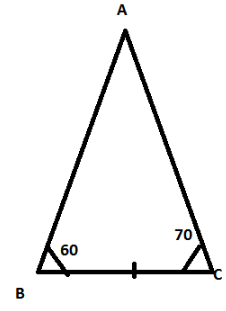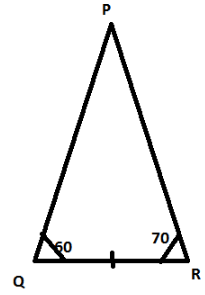#### Question 4:

In ∆ ABC, it is known that ∠B = ∠C. Imagine you have another copy of ∆ ABC
(i) Is ∆ ABC ≅ ∆ ACB?
(ii) State the three pairs of matching parts you have used to answer (i).
(iii) Is it true to say that ABAC?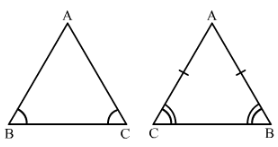(i) Yes△ABC ≅△ACB
(ii) We have used ∠ABC=∠ACB and ∠ACB =∠ABC again
Also BC = CB

(iii) Yes, it is true to say that AB = AC since ∠ABC=∠ACB

#### Question 5:

In Fig. 38, AX bisects ∠BAC as well as ∠BDC. State the three facts needed to ensure that ∆ ABD ≅ ∆ ACD.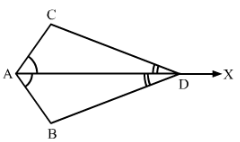Therefore, by ASA, △ACD≅△ABD

Question 6:

In Fig. 39, AOOB and ∠A = ∠B.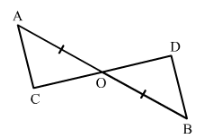(i) Is ∆ AOC ≅ ∆ BOD?
(ii) State the matching pair you have used, which is not given in the question.
(iii) Is it true to say that ∠ACO = ∠BDO?

We have
∠OAC =∠OBD, AO = OBAlso, ∠AOC = ∠BOD (Opposite angles on same vertex)

Therefore, by ASA △AOC ≅△BOD

97 docs

,

,

,

,

,

,

,

,

,

,

,

,

,

,

,

,

,

,

,

,

,

,

,

,

,

,

,

,

,

,

;